# Collocation method

A projection method for solving integral and differential equations in which the approximate solution is determined from the condition that the equation be satisfied at certain given points. For example, for the approximate solution of the integral equation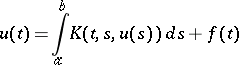one chooses a certain-parameter family of functions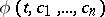and certain points (collocation knots, collocation nodes)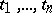on the interval. The approximate solution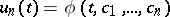is determined from the conditionswhich represent a system ofequations in the unknowns. If the equation is linear and the approximate solution is sought in the form of a linear combinationof the given (so-called coordinate) functions, then the system of equations inwill also be linear.

## Convergence of the collocation method for linear boundary value problems.

Suppose that foron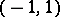the following boundary value problem is posed:(1)(2)

One looks for an approximate solution of this problem in the form where theare polynomials of degreesatisfying the boundary conditions (2). The coefficientsare determined from the linear system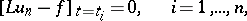(3)

with Chebyshev nodes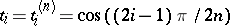,. The following theorem  holds. Suppose that the functionsand,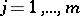, are continuous on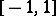and that the boundary value problem (1), (2) has a unique solution. Then there exists ansuch that for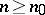the system (3) is uniquely solvable and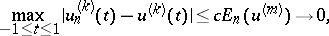where,Similar results hold (see ) if the nodes are roots of orthogonal polynomials with respect to some weight function. For equally-spaced nodes the above method diverges. Effective computational schemes with coordinate spline functions have also been developed (see , , ).